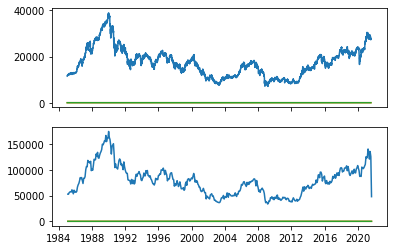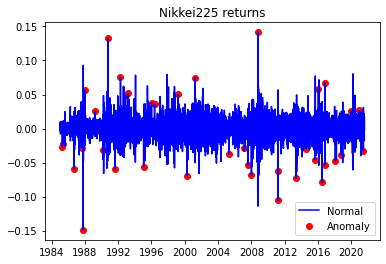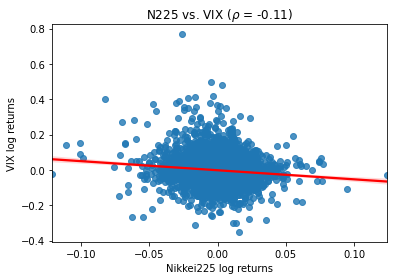# Japan Stock Price Nikkei 225 Analysis in Python

## What is Nikkei 225?

Nikkei 225 is a stock market index for the Tokyo Stock Exchange.
Analyzing the Nikkei 225, Japan's most prominent stock market index, using Python involves collecting historical price data, performing data analysis, and creating visualizations to gain insights into the index's performance. Here's a step-by-step guide on how to conduct Nikkei 225 price analysis in Python:

Import Libraries:
Start by importing the necessary Python libraries for data manipulation, analysis, and visualization. Commonly used libraries include pandas, numpy, and matplotlib. You may also need a financial data API like yfinance to fetch historical data:

Python
Copy code
import pandas as pd
import numpy as np
import matplotlib.pyplot as plt
import yfinance as yf
Data Retrieval:
Use the yfinance library to fetch historical Nikkei 225 data. Specify the start and end dates for the data you want to analyze:

Python
Copy code
Data Exploration:
Explore the fetched data to understand its structure and contents. You can use functions like head(), tail(), describe(), and info():

Python
Copy code
Data Visualization:
Create visualizations to analyze the historical performance of the Nikkei 225. Common visualizations include line charts to visualize the index's price movements:

Python
Copy code
plt.figure(figsize=(12, 6))
plt.title('Nikkei 225 Index Price')
plt.xlabel('Date')
plt.ylabel('Price')
plt.legend()
plt.show()
Technical Analysis (Optional):
Perform technical analysis by calculating and visualizing technical indicators like moving averages, relative strength index (RSI), and MACD. Popular Python libraries like ta-lib can help with these calculations.

Statistical Analysis (Optional):
Conduct statistical analysis to calculate summary statistics, volatility measures, and correlations with other assets. You can use Numpy and Pandas for these calculations.

Sentiment Analysis (Optional):
Consider incorporating sentiment analysis of news articles or social media data related to the Japanese stock market to understand market sentiment's impact on the Nikkei 225.

Fundamental Analysis (Optional):
Analyze fundamental factors affecting the Japanese economy, such as GDP growth, inflation rates, and interest rates, which can influence Nikkei 225's performance.

Prediction and Forecasting (Optional):
You can use time series forecasting techniques like ARIMA or machine learning models to make predictions about future Nikkei 225 movements.

Risk Management and Decision Making:
Based on your analysis, formulate investment strategies, set risk management parameters, and make informed investment decisions regarding Nikkei 225 or Japanese stocks.

Keep your analysis up to date with the latest data to adapt to changing market conditions and make timely decisions.

Remember that investing in stock markets carries risks, and it's crucial to do thorough research, consider the political and economic climate, and potentially consult with financial experts before making any investment decisions based on your analysis of the Nikkei 225 or any other stock index.
How to get  Nikkei 225 stock price?
We can get data from yahoo finance,quandai,wiki etc.
You can get data through python code.
import yfinance as yf
import numpy as np
import pandas as pd
import seaborn as sns
import scipy.stats as scs
import statsmodels.api as sm
import statsmodels.tsa.api as smt
import matplotlib.pyplot as plt
Code in python
start='1985-01-01',
end='2021-08-04',
progress=False)

### How to convert the Adj Close price?

#### Getting simple and log return

df[['simple_rtn','log_rtn']].tail(20)
simple_rtnlog_rtn
Date
2021-07-060.0015740.001573
2021-07-07-0.009645-0.009692
2021-07-08-0.008775-0.008814
2021-07-09-0.006317-0.006337
2021-07-120.0224980.022249
2021-07-130.0052230.005210
2021-07-14-0.003822-0.003829
2021-07-15-0.011514-0.011581
2021-07-16-0.009760-0.009808
2021-07-19-0.012511-0.012590
2021-07-20-0.009568-0.009614
2021-07-210.0058360.005819
2021-07-260.0103560.010303
2021-07-270.0049200.004908
2021-07-28-0.013892-0.013989
2021-07-290.0072790.007252
2021-07-30-0.017955-0.018118
2021-08-020.0182320.018068
2021-08-03-0.005010-0.005023
2021-08-04-0.002089-0.002091

#### How to get realised volatility in python?

def realized_volatility(x):
return np.sqrt(np.sum(x**2))
df_rv = df.groupby(pd.Grouper(freq='M')).apply(realized_volatility)
df_rv.rename(columns={'log_rtn''rv'}, inplace=True)
df_rv.rv = df_rv.rv * np.sqrt(12)
fig, ax = plt.subplots(21, sharex=True)
ax.plot(df)
ax.plot(df_rv)
[<matplotlib.lines.Line2D at 0x7f7559778410>,
<matplotlib.lines.Line2D at 0x7f753b5b9dd0>,
<matplotlib.lines.Line2D at 0x7f753b5b9fd0>]fig, ax = plt.subplots(31, figsize=(2420), sharex=True)
ylabel = 'Stock price ($)') df.simple_rtn.plot(ax=ax) ax.set(ylabel = 'Simple returns (%)') df.log_rtn.plot(ax=ax) ax.set(xlabel = 'Date', ylabel = 'Log returns (%)') df_rolling = df[['simple_rtn']].rolling(window=21) \ .agg(['mean''std']) df_rolling.columns = df_rolling.columns.droplevel() df_outliers = df.join(df_rolling) def indentify_outliers(rown_sigmas=3): x = row['simple_rtn'] mu = row['mean'] sigma = row['std'] if (x > mu + 3 * sigma) | (x < mu - 3 * sigma): return 1 else: return 0 #### How to plot outliers in Python? df_outliers['outlier'] = df_outliers.apply(indentify_outliers, axis=1) outliers = df_outliers.loc[df_outliers['outlier'] == 1, ['simple_rtn']] fig, ax = plt.subplots() ax.plot(df_outliers.index, df_outliers.simple_rtn, color='blue', label='Normal') ax.scatter(outliers.index, outliers.simple_rtn, color='red', label='Anomaly') ax.set_title("Nikkei225 returns") ax.legend(loc='lower right') <matplotlib.legend.Legend at 0x7f75364b5e50>r_range = np.linspace(min(df.log_rtn), max(df.log_rtn), num=1000) mu = df.log_rtn.mean() sigma = df.log_rtn.std() norm_pdf = scs.norm.pdf(r_range, loc=mu, scale=sigma) fig, ax = plt.subplots(12, figsize=(168)) # histogram sns.distplot(df.log_rtn, kde=False, norm_hist=True, ax=ax) ax.set_title('Distribution of Nikkei225 returns', fontsize=16) ax.plot(r_range, norm_pdf, 'g', lw=2, label=f'N({mu:.2f}{sigma**2:.4f})') ax.legend(loc='upper left'); #### How to plot Nikkei225 and vix in Python? df = yf.download(['^N225''^VIX'], start='1985-01-01', end='2021-07-28', progress=False) df = df[['Adj Close']] df.columns = df.columns.droplevel(0) df = df.rename(columns={'^N225''Nikkei225''^VIX''vix'}) df['log_rtn'] = np.log(df.Nikkei225 / df.Nikkei225.shift(1)) df['vol_rtn'] = np.log(df.vix / df.vix.shift(1)) df.dropna(how='any', axis=0, inplace=True) corr_coeff = df.log_rtn.corr(df.vol_rtn) ax = sns.regplot(x='log_rtn', y='vol_rtn', data=df, line_kws={'color''red'}) ax.set(title=f'N225 vs. VIX ($\\rho$= {corr_coeff:.2f})', ylabel='VIX log returns', xlabel='Nikkei225 log returns') [Text(0, 0.5, 'VIX log returns'), Text(0.5, 0, 'Nikkei225 log returns'), Text(0.5, 1.0, 'N225 vs. VIX ($\\rho\$ = -0.11)')]